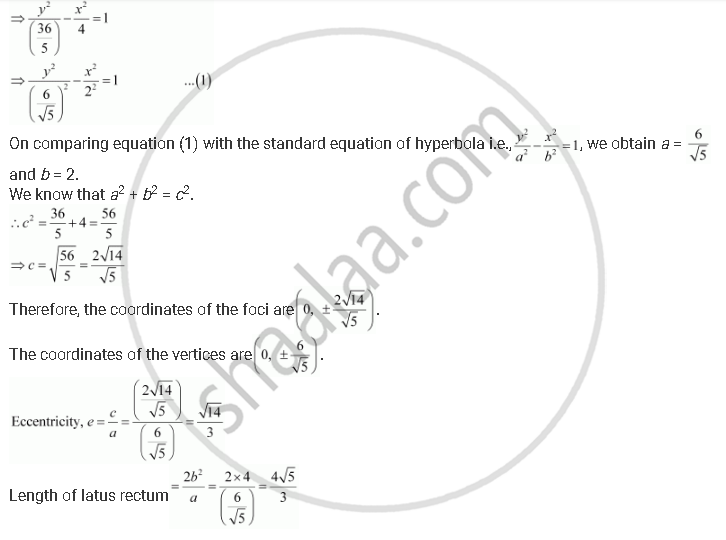Share

# Find the Coordinates of the Foci and the Vertices, the Eccentricity, and the Length of the Latus Rectum of the Hyperbola 5y2 – 9x2 = 36 - Mathematics

#### Question

Find the coordinates of the foci and the vertices, the eccentricity, and the length of the latus rectum of the hyperbola 5y2 – 9x2 = 36

#### Solution

The given equation is 5y2 – 9x2 = 36.Is there an error in this question or solution?